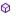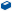Press on the image to return to the main documentation page.

# MatrixOp

MatrixOp

### MatrixOp

The library provides mathematical operations which involve matrices and scalars.
Names used here are :
Matrix is equivalent to Array of two dimensions.
The size is defined by number of rows (the first argument of the array)
and number of columns (second argument).
Vector is an Array of one dimension.
Scalar is a single number.
The library includes:
Operations of a Scalar and a Matrix
Operations between two matrices
Operations on a single matrix
There are no limitations on the size of the matrices or vectors.
There is no checking for incorrect dimensions of the matrices.
If not otherwise defined, all methods return 1 at completion.
The Library makes use of BigDecimal numbers to improve accuracy
by minimizing truncation errors.

None

#### Members:AddM (Rows As Int, Cols As Int, MatrixA(,) As Double, MatrixB(,) As Double, MatrixC(,) As Double) As DoubleAddS (Rows As Int, Cols As Int, MatrixA(,) As Double, Scalar As Double, MatrixC(,) As Double) As DoubleClear (Rows As Int, Cols As Int, MatrixA(,) As Double) As DoubleCopyM (Rows As Int, Cols As Int, MatrixA(,) As Double, MatrixC(,) As Double) As DoubleDeterminant (Size As Int, MatrixA(,) As Double) As DoubleDivS (Rows As Int, Cols As Int, MatrixA(,) As Double, Scalar As Double, MatrixC(,) As Double) As DoubleDotV (Length As Int, VectorA() As Double, VectorB() As Double) As DoubleInverse (Size As Int, MatrixA(,) As Double, MatrixC(,) As Double) As DoubleMulM (Rows As Int, Cols As Int, MatrixA(,) As Double, MatrixB(,) As Double, MatrixC(,) As Double) As DoubleMulS (Rows As Int, Cols As Int, MatrixA(,) As Double, Scalar As Double, MatrixC(,) As Double) As DoubleRoundby As IntSetRound (Roundto As Int)SolveM (Size As Int, MatrixA(,) As Double, Cols As Int, MatrixB(,) As Double, MatrixX(,) As Double) As DoubleSolveV (Size As Int, MatrixA(,) As Double, VectorB() As Double, VectorX() As Double) As DoubleSubM (Rows As Int, Cols As Int, MatrixA(,) As Double, MatrixB(,) As Double, MatrixC(,) As Double) As DoubleSubS (Rows As Int, Cols As Int, MatrixA(,) As Double, Scalar As Double, MatrixC(,) As Double) As DoubleTranspose (Rows As Int, Cols As Int, MatrixA(,) As Double, MatrixC(,) As Double) As Double

#### Members description:

#####AddM (RowsAsInt, ColsAsInt, MatrixA(,) AsDouble, MatrixB(,) AsDouble, MatrixC(,) AsDouble) AsDouble
Rows and Cols refer to the rows and columns of the three matrices,
MatrixC is the result: MatrixC = MatrixA + MatrixB.
#####AddS (RowsAsInt, ColsAsInt, MatrixA(,) AsDouble, ScalarAsDouble, MatrixC(,) AsDouble) AsDouble
Adds a Scalar to every element of MatrixA.
Rows and Cols refer to the rows and columns of MatrixA
and MatrixC which is the result : MatrixC = MatrixA + Scalar.
#####Clear (RowsAsInt, ColsAsInt, MatrixA(,) AsDouble) AsDouble
Put zeroes to all elements of MatrixA.
This method is the only method that actually changes
the referenced matrix.
#####CopyM (RowsAsInt, ColsAsInt, MatrixA(,) AsDouble, MatrixC(,) AsDouble) AsDouble
Copies MatrixA to MatrixC,
both have to be with the same dimensions Rows and Cols.
#####Determinant (SizeAsInt, MatrixA(,) AsDouble) AsDouble
The Determinant of the square matrix MatrixA with dimension Size
is returned as double by this method.
#####DivS (RowsAsInt, ColsAsInt, MatrixA(,) AsDouble, ScalarAsDouble, MatrixC(,) AsDouble) AsDouble
Divides every element of MatrixA by a Scalar.
Rows and Cols refer to the rows and columns of MatrixA
and MatrixC which is the result:
MatrixC = MatrixA/Scalar .
#####DotV (LengthAsInt, VectorA() AsDouble, VectorB() AsDouble) AsDouble
Dot-multiply two vectors
(each element is multiplied by the parallel element of the other vector
and the result is the sum of all multiplied pairs).
Length refer to the length of the two vectors which must be the same.
The result is returned by the method.
#####Inverse (SizeAsInt, MatrixA(,) AsDouble, MatrixC(,) AsDouble) AsDouble
MatrixA is a square matrix with dimension Size.
MatrixC is the inverse of MatrixA.
Returns 0 if the determinant is 0
(hence there is no inverse matrix).
#####MulM (RowsAsInt, ColsAsInt, MatrixA(,) AsDouble, MatrixB(,) AsDouble, MatrixC(,) AsDouble) AsDouble
Vector-Multiply two matrices.
Rows and Cols refer to the rows and columns of MatrixA,
MatrixB has to be with Cols rows and Rows columns,
MatrixC is the result and is of dimension Rows by Rows.
MatrixC = MatrixA X MatrixB.
#####MulS (RowsAsInt, ColsAsInt, MatrixA(,) AsDouble, ScalarAsDouble, MatrixC(,) AsDouble) AsDouble
Multiplies every element of MatrixA by a Scalar.
Rows and Cols refer to the rows and columns of MatrixA
and MatrixC which is the result :
MatrixC = Scalar * MatrixA.
#####SolveM (SizeAsInt, MatrixA(,) AsDouble, ColsAsInt, MatrixB(,) AsDouble, MatrixX(,) AsDouble) AsDouble
Solves a set of linear equations of the form AX = B,
where A is represented by MatrixA, B is represented by MatrixB
and the unknown matrix X is the result MatrixX.
Returns 0 if the determinant is 0 (hence there is no solution).
Size defines the size of the square MatrixA,
Cols define the number of columns in MatrixB and in MatrixX.
#####SolveV (SizeAsInt, MatrixA(,) AsDouble, VectorB() AsDouble, VectorX() AsDouble) AsDouble
Solves a set of linear equations of the form AX = B,
where A is represented by MatrixA, B is represented by VectorB
and the unknowns vector X is the result VectorX.
Returns 0 if the determinant is 0 (hence there is no solution).
Size defines the size of the square MatrixA,
and the length of VectorB and VectorX.
#####SubM (RowsAsInt, ColsAsInt, MatrixA(,) AsDouble, MatrixB(,) AsDouble, MatrixC(,) AsDouble) AsDouble
Adds a Scalar to every element of MatrixA.
Rows and Cols refer to the rows and columns of MatrixA
and MatrixC which is the result : MatrixC = MatrixA + Scalar.
#####SubS (RowsAsInt, ColsAsInt, MatrixA(,) AsDouble, ScalarAsDouble, MatrixC(,) AsDouble) AsDouble
Subtracts a Scalar from every element of MatrixA.
Rows and Cols refer to the rows and columns of MatrixA
and MatrixC which is the result: MatrixC = MatrixA - Scalar.
#####Transpose (RowsAsInt, ColsAsInt, MatrixA(,) AsDouble, MatrixC(,) AsDouble) AsDouble
MatrixC is the Transpose of MatrixA which is of
Rows rows and Cols columns.
MatrixC dimensions are Cols rows and Rows columns.
Top